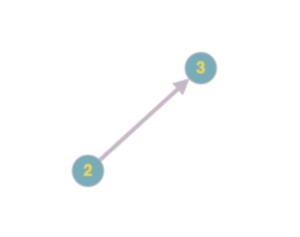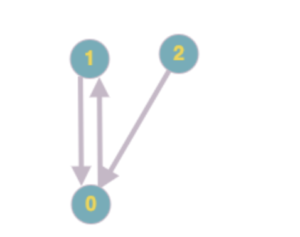# [Leetcode] Course Schedule II

What is topological sort? You simply keep picking nodes with no incoming edges (or with in-order 0).No valid solution? You might encounter situations where none of the nodes (yet to be processed) have an inorder of 0. This means that the graph does not have a valid topological sort (and we need to account for that). Here is an example. After picking ‘2’, you’re stuck with this 1-0, 0-1 cycle where inorder’s for both nodes are 1.Basic Idea:

• Create a dependency graph using the dependencies. The topological sort of this graph is your solution. Represent your graph using a HashMap (node_id -> [ child_id1, child_id2, .. child_idN ]).
• Use a Q to keep track of nodes with inorder 0. Don’t loop through all nodes to check for nodes with inorder 0 (except for the first time). Remember, at every step the only nodes that have a potential to have inorder 0 are the children of the last picked node!
• Finally, if you haven’t processed all the nodes (which means there is no valid topological sort) remember to return empty array.
```class Solution { public int[] findOrder(int numCourses, int[][] prerequisites) { Map<Integer, List<Integer>> graph = new HashMap<Integer, List<Integer>>(); int inorders[] = new int[numCourses]; int order[] = new int[numCourses]; int orderIndex = 0;   // build graph for ( int i = 0 ; i < prerequisites.length ; i++ ) { List<Integer> children = graph.getOrDefault(prerequisites[i], new ArrayList<Integer>()); children.add(prerequisites[i]); graph.put(prerequisites[i], children); inorders[prerequisites[i]]++; }   // have q keep track of all nodes with inorder = 0 // we will keep adding nodes to this q as and when thier inorder get to 0 Queue<Integer> q = new LinkedList<Integer>(); for ( int i = 0 ; i < inorders.length ; i++ ) { if (inorders[i] == 0) { q.add(i); } }   // pick nodes with zero indegree and add to list // make sure we update (decrement) indegrees of child nodes while ( !q.isEmpty() ) { int nextNode = q.remove(); List<Integer> children = graph.get(nextNode); if ( children != null ) { for ( Integer child : children ) { inorders[child]--; if (inorders[child] == 0) { q.add(child); } } } order[orderIndex++] = nextNode; }   // For cases where there are cycles in graphs we wont get a topological sort for all the nodes. T his is a way to detect that! // Examples: [[0,1],[1,0]], [[1,0],[1,2],[0,1]] if ( orderIndex < numCourses ) return new int;   return order; } }```